# Accuplacer Advanced Algebra and Functions Practice Test 2022

Accuplacer Advanced Algebra and Functions (AAF) Practice Test 2022: Download our next generation Accuplacer Advanced Algebra and Functions Placement Practice Test PDF. The Advanced Algebra and Functions Test includes the most sophisticated math found on the ACCUPLACER.

DIRECTIONS: This test contains items that measure your ability to do math at an introductory college level. Choose the best answer to each question. Feel free to use scrap paper. You may not use a calculator. However, with some problems with the computer-based ACCUPLACER, you may be given access to an on-screen calculator with limited functionality.

## Accuplacer Advanced Algebra and Functions Test

 Name of the Test Accuplacer Practice Test Administrated by College Board Total questions 30 Question format multiple-choice questions Test subjects Advanced Algebra and Functions (AAF)

Q1. If \frac1a + \frac{1}{2a} = b , what is the value of a in terms of b?

• (A) \frac{2}{3b}
• (B) \frac{3}{2b}
• (C) \frac{2b}{3}
• (D) \frac{3b}{2}

Q2. (2x – 3) (x² + 4x – 5) is equivalent to which of the following expressions?

• (A) 2x³ + 5x² + 2x + 15
• (B) 2x³ – 5x² – 2x + 15
• (C) 2x³ + 5x² – 2x + 15
• (D) 2x³ – 5x² + 2x + 15

Q3. In the following figure, lines AB and CD are parallel, with AD and BC intersecting at E. If the lengths of AE, BE, and EC are 2, 4, and 5 respectively, what is the length of ED?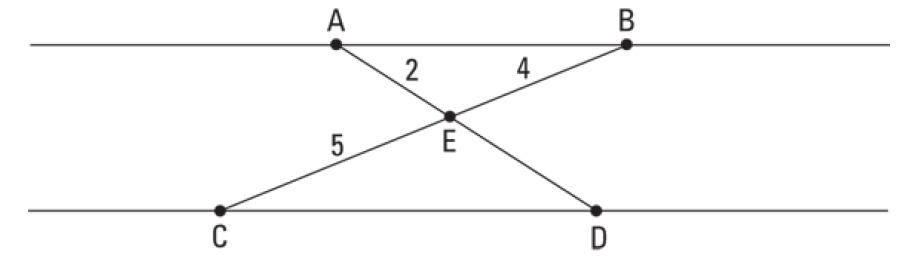• (A) 2.4
• (B) 2.5
• (C) 2.6
• (D) 2.8

Q4. If f(x) = x² -4x and g(x) = 3x + 6, what is the value of \frac{f(6)}{g(-4)} = ?

• (A) 2
• (B) 1
• (C) -1
• (D) -2

Q5. What is the equation of the line that is parallel to the following line and passes through the origin?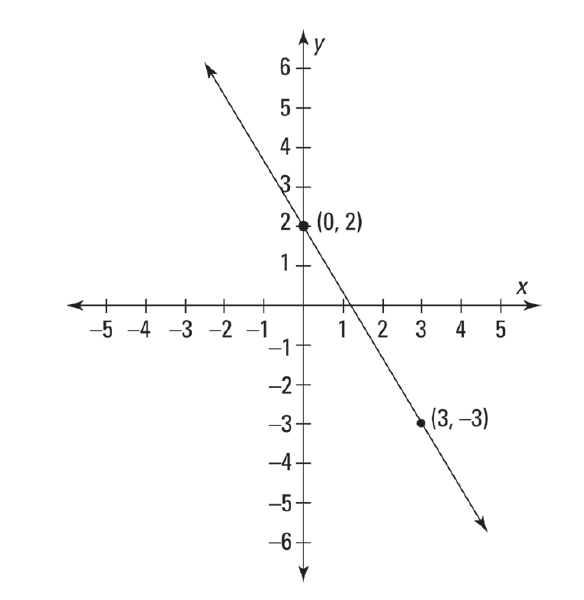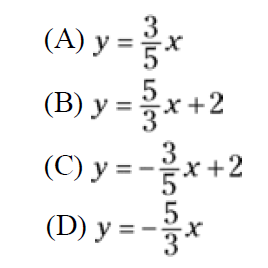Q6. All of the following are functions EXCEPTQ7. If a and b are the two solutions to the equation 2x² – 14x = -20, which of the following is a possible value of a-b?

• (A) 3
• (B) 5
• (C) 7
• (D) 10

Q8.Which of the following equations is the function shown in this xy graph?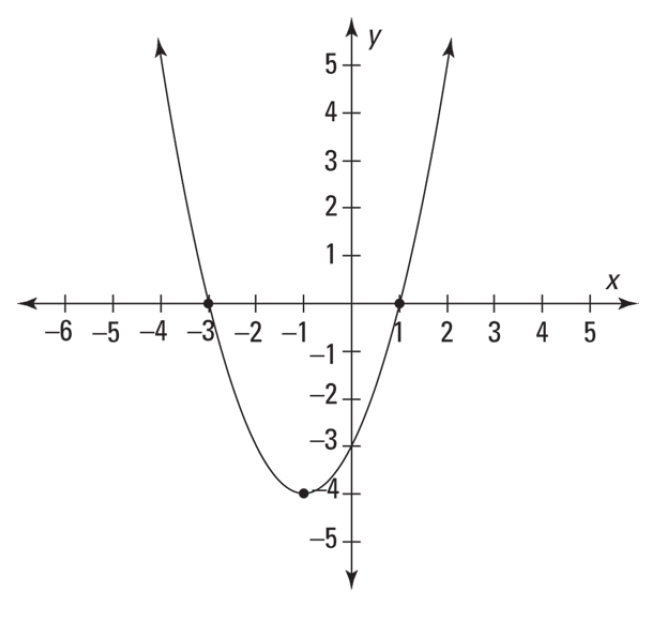• (A) y= (x + 1)(x + 3)
• (B) y= (x + 1)(x – 3)
• (C) y= (x + 1)² -4
• (D) y= (x – 1)² -4

Q9. Points A and B are two points on the circle centered at O, shown here. If OB= 4 and the measure of angle AOB is 40 degrees, what is the arc length AB?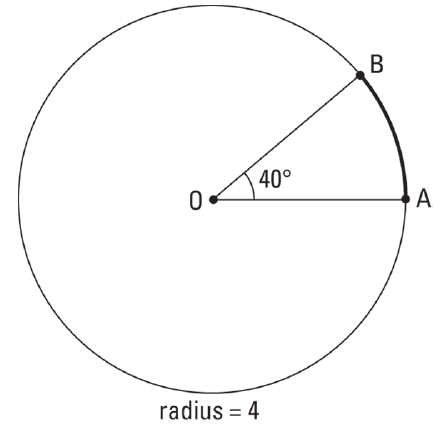• (A) \frac{2π}{9}
• (B) \frac{4π}{9}
• (C) \frac{8π}{9}
• (D) \frac{16π}{9}

Q10. Jonathan invested \$2000 in a CD that paid 4% interest compounded annually. He used the equation f(t)=2000(n)t to calculate the amount his CD will be worth in t years. Which of the following values should he use for n?

• (A) 0.04
• (B) 0.4
• (C) 1.04
• (D) 1.4

Q11. What is the value of x if \frac{x+2}{x-3} = \frac{x-5}{x-1} ?

• (A) \frac{13}{7}
• (B) \frac{13}{9}
• (C) \frac{17}{7}
• (D) \frac{17}{9}

Q12. Which value(s) are excluded from the domain of the function fx = \frac{1}{x² - 10x + 25} ?

• (A) 5
• (B) -5
• (C) 5 and -5
• (D) No values are excluded.

Q13. The equation \frac{x - 4}{4} = \frac{2}{x - 6} has two possible values for x. What is the sum of these two values?

• (A) 8
• (B) 10
• (C) 12
• (D) 16

Q14. If log611x = 2, then x equals

• (A) \frac{11}{36}
• (B)  \frac{11}{64}
• C) \frac{36}{11}
• (D) \frac{64}{11}

Q15. In triangle XYZ, angle Y is a right angle if sin X= \frac{7}{25} then sin Z =?

• (A) \frac{7}{24}
• (B) \frac{24}{25}
• C) \frac{24}{7}
• (D) \frac{25}{7}

Q16. Which of the following is the equation for this circle?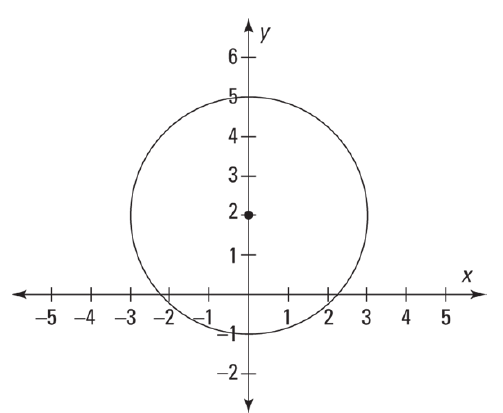• (A) (x-2)² + y² =3
• (B) x² + (y+2)² =3
• (C) (x+2)² + y² =9
• (D) x² + (y-2)² =9

Q17. If f(x)=x² – 4x, what is the value of 2f(a-1)?

• (A) 2a² + 8a – 6
• (B) 2a² – 12a – 6
• (C) 2a² – 8a + 10
• (D) 2a² – 12a + 10

Q18. \sqrt{x² -1}-2=x Solve for x.

• (A) \frac{4}{5}
• (B) \frac{5}{4}
• C) - \frac{4}{5}
• (D) - \frac{5}{4}

Q19. if 125 x-1 = \sqrt{5}x+1, what is the value of x?

• (A) \frac{5}{7}
• (B) \frac{7}{5}
• C) - \frac{5}{7}
• (D) - \frac{7}{5}

Q20. if x-y = 3 and x² – y² =15 then x³ – y³ =?

• (A) 27
• (B) 63
• (C) 75
• (D) 255

Q21. if f(x) = x² – 7, what is the value of f(3)?

• (A) 2
• (B) -2
• (C) 22
• (D) -22

Q22. Which of the following is true of the end behavior of polynomial 3x5 – 5x3 – 7x?

• (A) It’s positive for both very large and very small values of x.
• (B) It’s negative for both very large and very small values of x.
• (C) It’s positive for very large values of x and negative for very small values of x.
• (D) It’s negative for very large values of x and positive for very small values of x.

Q23. Which of the following functions includes the point (0, -9) when graphed on the xy-plane?

• (A) y = x3 – 5x2 -x + 9
• (B) y = -9x3 + 4x2 – 6x – 2
• (C) y = 6x3 – 11x2 + 8x – 9
• (D) y = 3x3 + 4x2 -x + 9x

Q24. Which of the following is a root of the function?

y = (x+3)(x-1)(2x-5)

• (A)   \frac{2}{5}
• (B)   \frac{5}{2}
• (C) - \frac{2}{5}
• (D) - \frac{5}{2}